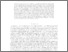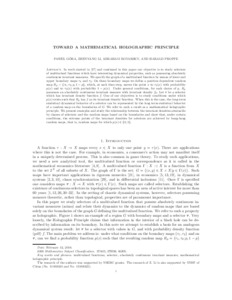Title:

# Toward a Mathematical Holographic Principle

Góra, Paweł, Li, Zhenyang, Boyarsky, Abraham and Proppe, Harald (2014) Toward a Mathematical Holographic Principle. Journal of Statistical Physics, 156 (4). pp. 775-799. ISSN 0022-4715Preview Text (application/pdf) Selections_and_Random_Maps_Revisedv11.pdf - Accepted Version Available under License Spectrum Terms of Access. 363kB

Official URL: http://dx.doi.org/10.1007/s10955-014-1029-4

## Abstract

Let $X$ and $Y$\ be given sets of real line and let $T(x)\subset Y$ for each $x\in X.$ Consider the Cartesian product $G=\Pi _{x\in X}T(x).$ The elements of this
products are maps $\tau :X\rightarrow Y$ such that $\tau (x)\in T(x)$ for each $x\in X$, and are called selectors of $T.$ The main problem pertaining to selectors is to determine under which conditions on $T$ there exists a
selector having desired topological properties. In work started in \cite{GLB13} and continued in this paper our objective is to study selectors which have interesting dynamical properties, such as possessing absolutely continuous invariant measures. We shall specify $G$ by means of fixed lower and upper boundary maps $\tau _{1}$ and $\tau _{2}.$ On these boundary maps we define a position dependent random map $R_{p}=\{\tau _{1},\tau _{2};p,1-p\},$ which, at each time step, moves the point $x$ to $\tau _{1}(x)$ with probability $p(x)$ and to $\tau _{2}(x)$ with probability $1-p(x)$. Under
general conditions, for each choice of $p(x),$ $R_{p\text{ }}$possesses an absolutely continuous invariant measure with density $f_{p}.$ We refer to this function as an invariant density. Let $\tau$ be a selector which has invariant density function $f.$ One of our objectives is to study conditions under which $p(x)$ exists such that $R_{p}$ has $f$ as its invariant density
function. When this is the case we claim the long term statistical dynamical behavior of a selector (image inside $G$) can be represented by the long term statistical behavior of a random map on the boundaries of $G.$ We refer to such a result as a mathematical holographic principle. We present examples and study the relationship between the invariant densities attainable by classes of selectors and the random maps based on the
boundaries. We show that under certain conditions the extreme points of the invariant densities for selectors are achieved by bang-bang random maps, that is, random maps for which $p(x)\in \{0,1\}.$

Divisions: Concordia University > Faculty of Arts and Science > Mathematics and Statistics Article Yes Góra, Paweł and Li, Zhenyang and Boyarsky, Abraham and Proppe, Harald Journal of Statistical Physics 2014 10.1007/s10955-014-1029-4 980103 PAWEL GORA 25 Jun 2015 16:04 18 Jan 2018 17:50
All items in Spectrum are protected by copyright, with all rights reserved. The use of items is governed by Spectrum's terms of access.

Repository Staff Only: item control pageResearch related to the current document (at the CORE website)
Back to top